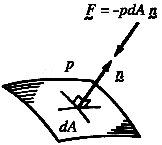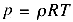Pressure

## Pressure

Pressure is a stress. It is a scalar given by the magnitude of the force per unit area. In a gas, it is the force per unit area exerted by the change of momentum of the molecules impinging on the surface. We know from Newton's second law that a net resultant force will cause a change of momentum in a body, and that the rate of change of momentum is equal to the applied force. It is a vector relationship, so that even if the magnitude of the momentum is unchanged, a change in the direction of motion requires a resultant force. The impact of a gas molecule on a solid surface is an elastic impact so that its momentum magnitude and energy are conserved. However, because its direction of motion changes on impact, a resultant force must have been exerted by the solid surface on the gas molecule. Conversely, an equal but opposite force was exerted by the gas molecule on the solid surface. If we consider a very small area of the surface of the solid, so that over a short time interval \Delta t very few molecules collide with the solid over this area, then the force exerted by the gas molecules will vary sharply with time. When we consider a sufficiently large area, so that the number of collisions on the surface during the interval \Delta t is large, then the average force that acts on the solid surface by the molecules is constant. It is this force, acting on the surface of the solid, that we call the force due to pressure.

In practice, the area need only be larger than about 10 times (mean free path)^2, so that pressure is a continuum property, by which we mean to say that for areas of engineering interest, which are almost always much larger than areas measured in terms of the mean free path, the pressure does not have any measurable statistical fluctuations caused by molecular motions. We can make a distinction between microscopic properties and the macroscopic properties of a fluid, where the microscopic properties relate to the behavior on a molecular scale (that is, scales comparable to the mean free path), and the macroscopic properties pertain to the behavior on an engineering scale (that is, scales much larger than the mean free path).

### Pressure: direction of action

Consider the line of action of the force due to pressure. On a molecular level, a flat solid surface is never flat. However, it is flat on average, so that, on average, for each molecule that rebounds with some component of momentum along the surface, another rebounds with the same component of the momentum in the opposite direction. The average momentum of the molecules in the direction along the surface will not change during their impact with the surface. We expect, therefore, that the average force due to pressure (on a macroscopic scale) acts in a direction which is purely normal to the surface. Furthermore, since the momentum of the molecules is randomly distributed in space, the magnitude of the force due to pressure should be independent of the direction of the surface on which it acts. For instance, a thin flat plate in air will experience a zero resultant force due to air pressure since the forces due to pressure on its two sides have the same magnitude (the pressure is independent of the orientation of the surface on which it acts) and they point in opposite directions (each force acts normal to its own surface). We say that pressure is isotropic (based on Greek words, meaning equal in all directions, or more accurately in the present case, independent of direction).Figure 7. The vector force due to pressure acting on an element of surface.

### Pressure: transmission through a fluid

An important property of pressure is that it is transmitted through the fluid. When an inflated bicycle tube is pressed at one point, for example, the pressure increases at every other point in the tube. Measurements show that the increase is the same at every point and equal to the applied pressure. For example, if an extra pressure of 5 psi were suddenly applied at the tube valve, the pressure would increase at every point of the tube by exactly this amount. This property of transmitting pressure undiminished is a well established experimental fact, and it is a property possessed by all fluids. The transmission does not occur instantaneously, but at a rate that depends on the speed of sound in the medium and the shape of the container. The speed of sound is important because it measures the rate at which pressure disturbances propagate (sound is just a pressure disturbance travelling through a medium). The shape of the container is important because pressure waves refract and reflect of the walls of the container and this increases the distance and time the pressure waves need to travel. This phenomenon should be familiar to anyone who has experienced the imperfect acoustics of a poorly designed concert hall.

### Compressibility in Fluids

All fluids are compressible. However, under some range of conditions, it is often possible to make the approximation that a fluid is incompressible. Water, for example, only changes its volume very slightly under extreme pressure.

Gases are much more compressible. The compressibility of air, for example, is part of our common experience. By blocking off a bicycle pump and pushing down on the handle, we can easily decrease the volume of the air by 50%, so that its density increases by a factor of two (the mass of air is constant). If we assume that the temperature remains constant (somehow), we know from the perfect gas law,that the pressure must also increase by a factor of two. If the initial air was at atmospheric pressure, the pressure will rise by one atmosphere (14.696 psi, or 101,325 Pa). If we assume a pump diameter of 1.25 inch, then a force of 18.1 lbf will be required. This is not a large force, so that raising the pressure by two atmospheres above ambient pressure is easily done.

We call pressures relative to zero pressure absolute pressure. This is the pressure that appears in the perfect gas law. Pressures measured relative to atmospheric pressure are called gauge pressures. The pressure measured by the most common types of pressure instruments is a gauge pressure since these instruments indicate the pressure relative to atmospheric pressure. A tire gauge, for instance, measures the pressure in a tire over and above the local atmospheric pressure. A vacuum gauge, in contrast, will measure the pressure below atmospheric: in common parlance a ``vacuum'' is any pressure lower than the ambient atmospheric pressure.

Even though gases are much more compressible than liquids (by perhaps a factor of 10^4, or, four ``orders-of-magnitude''), if the pressures are small the changes in density are also small. For example, if a 1% change in density is tolerated, then at constant temperature we can allow a 1% change in pressure. For air this corresponds to a change in altitude of about 85 meters, so that for changes in height of the order of tall buildings we can usually neglect the compressibility of air. The compressibility of gases is also often discussed in terms of the parameter called the Mach number, which is the ratio of the fluid velocity to the speed of sound (the speed of sound for air at 20^o C is 343 m/s = 1,126 ft/s = 768 mph). As we shall see later, when a fluid increases its speed, there is a corresponding decrease in pressure. If the fluid velocity is small relative to the speed of sound (that is, the flow has a low Mach number), then the change in pressure when a fluid increases its velocity from zero to V is given by 1/2 the density times the velocity squared (in the absence of friction). This quantity is called the dynamic pressure:If we again use a 1% level as a tolerable change in density, then if the temperature remains constant the allowed change in pressure is also equal to 1% (the process is more likely to be isentropic, but that does not change the argument very much). This requires a dynamic pressure less than 1% of the ambient pressure, so that at sea level where the density of air is about 1.2 kg/m^3 we are limited to velocities less than 40 m/s (132 ft/s or 90 mph), which corresponds to a Mach number of about 0.12. When we consider the flow of air over bicycles, we can always assume that the fluid has a constant density, and that the Mach number is not important.

### Pressure and Lift

When we consider the forces acting on a wing, for example, the lift force is proportional to the average pressure difference acting over the lower and upper surface of the wing. This pressure difference is caused by the fact that the average velocity over the upper surface of the airfoil is somewhat greater than the average velocity over the lower surface (remember that a higher velocity corresponds to a lower pressure). The pressure differences are usually small, but wings have a large surface area so that the total lift force can be very large (a fully loaded 747 can weigh as much as 500,000 lbf). For low Mach number flow, the pressure difference and the lift force are proportional to the difference in the dynamic pressures between the upper and lower surfaces of the airfoil.